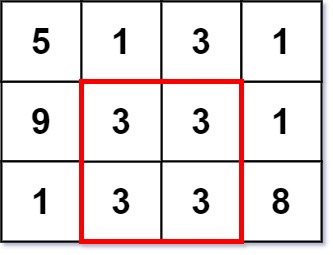Formatted question description: https://leetcode.ca/all/1895.html

# 1895. Largest Magic Square

Medium

## Description

A k x k magic square is a k x k grid filled with integers such that every row sum, every column sum, and both diagonal sums are all equal. The integers in the magic square do not have to be distinct. Every 1 x 1 grid is trivially a magic square.

Given an m x n integer grid, return the size (i.e., the side length k) of the largest magic square that can be found within this grid.

Example 1:Input: grid = [[7,1,4,5,6],[2,5,1,6,4],[1,5,4,3,2],[1,2,7,3,4]]

Output: 3

Explanation: The largest magic square has a size of 3.

Every row sum, column sum, and diagonal sum of this magic square is equal to 12.

• Row sums: 5+1+6 = 5+4+3 = 2+7+3 = 12
• Column sums: 5+5+2 = 1+4+7 = 6+3+3 = 12
• Diagonal sums: 5+4+3 = 6+4+2 = 12

Example 2:Input: grid = [[5,1,3,1],[9,3,3,1],[1,3,3,8]]

Output: 2

Constraints:

• m == grid.length
• n == grid[i].length
• 1 <= m, n <= 50
• 1 <= grid[i][j] <= 10^6

## Solution

The maximum possible size of a magic square is Math.min(m, n). Loop over all side lengths from Math.min(m, n) to 2, and find the largest side length such that there exists at least one magic square with the side length. Once such a side length is found, return the side length. Otherwise, return 1.

class Solution {
public int largestMagicSquare(int[][] grid) {
int m = grid.length, n = grid.length;
for (int side = Math.min(m, n); side > 1; side--) {
if (existMagicSquare(grid, m, n, side))
return side;
}
return 1;
}

public boolean existMagicSquare(int[][] grid, int m, int n, int side) {
int maxRow = m - side, maxColumn = n - side;
for (int i = 0; i <= maxRow; i++) {
for (int j = 0; j <= maxColumn; j++) {
if (isMagicSquare(grid, i, j, side))
return true;
}
}
return false;
}

public boolean isMagicSquare(int[][] grid, int startRow, int startColumn, int side) {
Set<Integer> set = new HashSet<Integer>();
int[] rowSums = new int[side];
int[] columnSums = new int[side];
int diagonal1 = 0, diagonal2 = 0;
for (int i = 0; i < side; i++) {
for (int j = 0; j < side; j++) {
rowSums[i] += grid[startRow + i][startColumn + j];
columnSums[j] += grid[startRow + i][startColumn + j];
if (i == j)
diagonal1 += grid[startRow + i][startColumn + j];
if (i + j == side - 1)
diagonal2 += grid[startRow + i][startColumn + j];
}
}
for (int i = 0; i < side; i++) {